mathscreative.com ad tags " amp " ShareThis BEGIN ShareThis END "". MATHEMATICS - LIFE SKILL, THINK OUT OF THE BOX: June 2020 "".

## Wednesday, June 24, 2020

### ABOUT AREA, ITS MPORTANCE, AREA OF DIFFERENT SHAPES AND INTERESTING QUESTIONS

AREA

The area is the space occupied within a shape. Perimeter is calculated as the boundary of a shape, while area is calculated as the space that is inside a shape. Area is always measured for two or more dimensional shape. One dimension is just the length, and no width, like this I
It is not possible to calculate its area.

IMPORTANCE OF AREA

Suppose the wall is to be covered with tiles, then how many tiles required, to cover that wall we must know the area of the wall and area of the tiles.

The cuboid is to be decorated with wall paper, meters or centimeters of wall paper needed to decorate it, we have to calculate its total surface area.

The window air conditioners are installed in windows, how much area of window the air conditioner would acquire, for that we have to ascertain the area of the air conditioner.

If we have to cover the table with the table cloth, then we must definitely know the area of the table, so that at least the table cloth is not short of table, but is more in area somewhat to the area of table, in order to fall on the sides of table also.

Area is of great use to architects, who prepare the maps of houses, residential apartments and other things, how much space to be given to kitchen, drawing room, how much margin to be given between two houses etc according to the total area available

WHY THE AREA OF RECTANGLE IS LENGTH X BREADTH AND OF SQUARE IS SIDE X SIDE

The area of rectangle is length X breadth, Length is 10 inches, and breadth is 8 inches, hence 10 X 8 = 80 square inches . The area of each square mat is 4 inches, and there are 20 square mats in the rectangle. So total area of 20 square mats is 20 X 4 = 80 inches, hence area of rectangle is length X breadth .

The area of square is side X side. Since each side in square is equal, so we denote it as side. So 10 X 10 = 100 square inches. There are 25 square mats in the square, therefore area of 25 square mats is 25 X 4 = 100 square inches. Thus area of square is side X side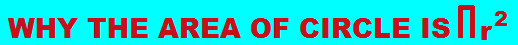WHY THE AREA OF TRIANGLE IS 1/2 X BASE X HEIGHT

REASON FOR THE AREA OF RHOMBUS - 1/2 X DIAGONAL 1 X DIAGONAL 2

AREA OF TRAPEZIUM IS SUM OF PARALLEL SIDES / 2 X HEIGHT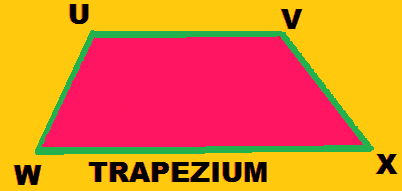We can divide this trapezium into two triangles which are WVX and UVW. Area of trapezium would be sum of these two triangles, since area of triangle is half X base X height, we need height so lets draw the height VY and as shown in this figure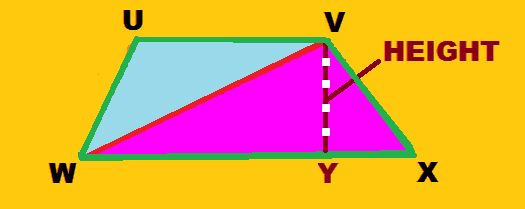Base of triangle WVX is WX and its height is VY, so area of this triangle is 1/2 X WX X VY. Now area of another triangle UVW, base of triangle UVW can VW or UV. But since the corresponding height of base UV is VY, we would take the base as UV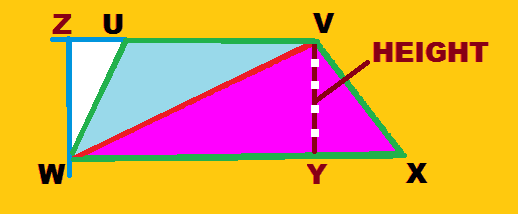If we draw a line WZ of same height as VY, which is perpendicular to base UV, we would know the height WZ, so area of triangle UVW would be 1/2 X UV X WZ. Now we know the area of both the triangles so we have to add, so it is 1/2 X WX X VY + 1/2 X UV X WZ. Since height VY and height WZ is equal we can also write it as
1/2 X WX X VY + 1/2 X UV X VY.
Hence we can take 1/2 and VY as common it is
1/2 X VY ( WX + UV )
So it can also be written as WX + UV X VY
2

WX and UV are parallel sides, that is the sides that would never meet, and when both are added it is their sum and VY is height, so area of trapezium is sum of parallel sides /2 X height

AREA OF REGULAR PENTAGON IS 5/2 X SIDE X RADIUS
The regular pentagon has five sides and five angles, where all the sides and all the angles are equal.  S is the side, in this pentagon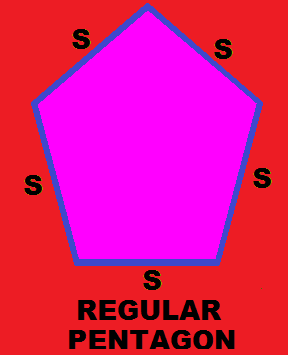We can divide this pentagon into five equal triangles, and if we know the area of one triangle we would calculate the area of pentagon by adding the area of a triangle five times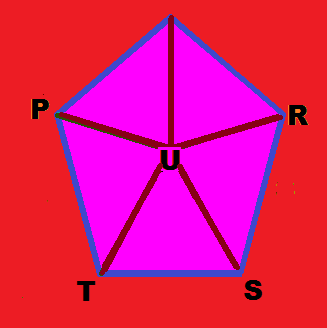So there are five triangles that is PUQ, QUR, RUS, SUT and TUP. Area of triangle is 1/2 X base X height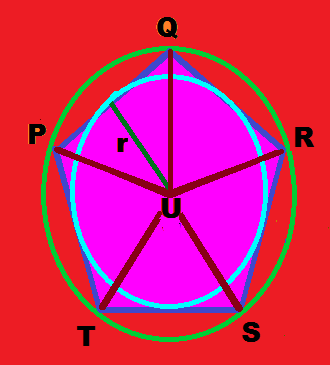r = radius .One outer circle, and one in circle can be drawn around this pentagon. So considering the area of triangle PUQ, PU is the base, and r is the radius of in circle drawn and also the corresponding height of the triangle PUQ. So area of the triangle PUQ is 1/2 X PU X R. PU is also the side of the pentagon. So area of triangle PUQ is 1/2 X Side X R. Since pentagon has five triangles its area is -
( 1/2 X Side X Radius ) 5 or it is 5/2 X Side X Radius.

AREA OF EQUILATERAL TRIANGLE

AREA OF REGULAR HEXAGON

AREA OF CUBOID

So B X H + B X H + B X L + B X L + H X L + H X L . Since B X H, B X L and H X L is repeated two times taking two as common area of cuboid is 2 ( BH + BL + HL )

AREA OF CYLINDER

AREA OF CONE

QUESTIONS BASED ON AREA

Ques – 1. The perimeter of the square is 50 meters. The perimeter of the rectangle is double of the perimeter of the square. If the length of the rectangle is 32 meters, how much is the area of the rectangle ?

(a) 576 sq meters

(b) 624 sq meters

(c) 792 sq meters

(d) 480 sq meters

Ques – 2. The base of an equilateral triangle is 60 centimeters. How much is its height and area ?

(a) Height 50.96 cm, area 1456.856 cm

(b) Height 51.96 cm, area 1558.845 cm

(c) Height 52.98 cm, area 1674.986 cm

(d) Height 51.26 cm, area 1550.856 cm

Ques – 3. This is a rectangular field. Inside this rectangular field a pavement is built leaving a margin of 5 meters. How much is the area of the pavement built ?

(a) 5,073.3 cm

(b) 4,073.3 cm

(c) 4,563.6 cm

(d) 5,346.4 cm

Ques – 4. The area of circle is 679.14 decimeters. How much is the circumference of one – third of the circle ?

(a) 305 cm

(b) 412 cm

(c) 308 cm

(d) 216 cm

Ques – 5. The piece of wood is in rectangular shape, and out of that a table is cut. How much is the area of the rectangular piece of wood left after cutting the table ?

(a) 30.46 m

(b) 40.36 m

(c) 36.36 m

(d) None of these

Ques – 6. The verandah is square shaped whose side is 27 meters. It is to be covered with tiles, whose length is 60 centimeters and breadth is half meters. The tiles are to be covered in the corridor excluding the corridor of 9 meters. How many tiles are required for covering the verandah ?

(a) 20 tiles

(b) 24 tiles

(c) 25 tiles

(d) 30 tiles

Ques – 7. The pentagon is a regular one. The side of its one of triangle is 10.5 centimeters, and its radius is 7 centimeters. How much is the area of the pentagon?

(a) 183.75 cm

(b) 178.80 cm

(c) 206.80 cm

(d) 189.70 cm

Ques – 8. Out of this square, the circle is cut. How much area is left after cutting ?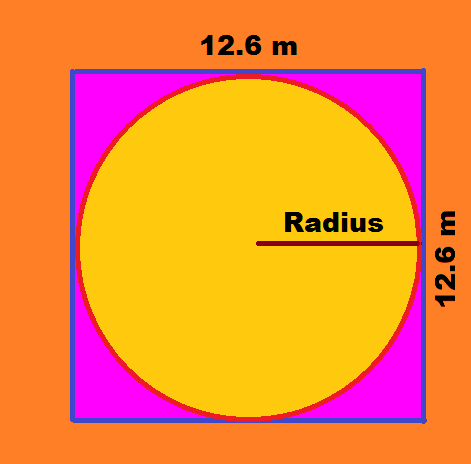(a) 35.04 m

(b) 42.06 m

(c) 36.04 m

(d) 34.02 m

Ques – 9. The breadth of the rectangle is 17.5 cm, and its  diagonal is 12 cm. How much is the area of the triangle, if a rectangle is divided into two equal parts ?

(a) 102 cm

(b) 105 cm

(c) 210 cm

(d) 150 cm

Ques – 10. The area of the cylinder is 1,925.77 cm square. How much is its height, if its radius is 14.35 cm ?

(a) 28.40 cm

(b) 21.70 cm

(c) 28.35 cm

(a) 85.016 inches

(b) 86.016 inches

(c) 80.616 inches

(d) 90.126 inches

Ques – 12. What is the area of the following figure ?

(a) 126.625 m

(b) 112.525 m

(c) 120.525 m

(d) 116.625 m

Ques - 13. How much is the area of cube if the area of its back face is 19.321 sq decameters ?

(a) 1,160.26 m

(b) 1,159.26 m

(c)  897.36 m

(d) 2,159.49 m

Ques - 14 .There are two regular hexagons. The area of first hexagon is 176.4031 sq cm, and area of second hexagon is 113.5154 sq cm. How much is the difference in side of two hexagons ?

(a) 1.63 cm

(b) 1.73 cm

(c) 2.64 cm

(d) 1.53 cm

Ques - 15 . The area of cuboid is 406.12 cm. If its length is 10.2 m, breadth is 6.4 m, calculate its height

(a) 8.2 m

(b) 8.3 m

(c) 10.5 m

(d) 9.5 m

Ques – 1.(a) The perimeter of the rectangle is double of the perimeter of the square, so perimeter of rectangle is 50 X 2 = 100 m. Length of rectangle is 32 meters, so its breadth is 50 – 32 = 18 meters. So area of the rectangle is 32 X 18 = 576 sq meters .

Ques - 2.(b) Height of an equilateral triangle is b√3/2, so its height is 60 √3/2 = 51.96 cm. Its area is b X b √3/4, hence area is 60 X 60 √3/4 = 1,558.845 cm

Ques – 3.(b) Margin of 5 meters is left all around. Hence breadth of the pavement is 90.5 – 10 = 80.5, and its length is 60.6 – 10 = 50.6, so its area is 80.5 X 50.6 = 4073.3 sq m

Ques - 4. (b) Height of an equilateral triangle is b√3/2, so its height is 60√3/2 = 51.96 cm. Its area is b X b √3/4, hence area is 60 X 60√3/4 = 1,558.845 cm

Ques - 5. (c) Area of the rectangular field is 7.8 X 7.2 = 56.16 meters. The table cut is in the shape of trapezium, so its area is 4.5 + 6.5 /2 X 3.6, hence area is 11/2 X 3.6 = 5.5 X 3.6 = 19.8 meters. Hence area left after cutting is 56.16 – 19.8 = 36.36 meters

Ques - 6. (b) Area of verandah is 27 X 27 = 729 sq meters Area to be excluded is 729 – 9 = 720 meters. Area of tile is 60 X 50 = 3,000 centimeters or 30 meters.  Hence number of tiles required is 720/30 = 24 tiles.  ( Half meter = 50 centimeters )

Ques - 7. (a) Area of pentagon is 5/2 X radius X base or side = 5/2 X 10.5 X 7 = 183.75 cm

Ques - 8. (d) Area of the square is 12.6 X 12.6 = 158.76. Area of the circle is 22/7 X 6.3 X 6.3 = 124.74. Hence area left after cutting the square is 158.76 – 124.74 = 34.02 meters

Ques - 9. (b) Breadth of the rectangle is 17.5 m, and its diagonal is 12 m, hence area of the triangle is 1/2 X 12 X 17.5 = 105 m .

Ques – 10.(c) Area of the cylinder  2 pie r ( r + h ),
2 X 22/7 X 14.35 ( 14.35 + h ) = 1925.77 cm
Hence 22 X 2.05 ( 14.35 + h ) = 1925.77 cm
So 45.10 ( 14.35 + h ) = 1925.77
14.35 + h = 1925.77/45.10
14.35 + h = 42.7
h = 42.7 – 14.35 = 28.35 cm

Ques - 11.(b) Area of the oval part of the table is 3.14 X 6 X 2.4 = 45.216 inches. Area of one leg is 8.5 X 1.2 = 10.2 inches, hence area of 4 legs is 10.2 X 4 = 40.8 inches. So area of the table is 45.216 + 40.8 = 86.016 inches

Ques - 12. (d) Area of the square ABCD is 5 X 5 = 25 m . Area of the rectangle EFGH is 8 X 5 = 40 m, FE is 8 m, as CF is 2 m, CD is 5 m, and DE is 1 m . Area of the triangle GHJ is 1/2 X 8 X 5.5 = 22 m . Area of the triangle GIJ is 1/2 X 10.5 X 4.5 = 23.625 m. Area of the triangle HJK is 1/2 X 4 X 3 = 6 m . Hence total area of the figure is 25 + 40 + 22 + 23.625 + 6 = 116.625 m

Ques - 13.(b) 19.321 decameters is 193.21 meters, as one decameter = 10 meters. Area of a face is a X a = 193.21,  hence area of the cube is 6 X 193.21 = 1,159.26 meters
Ques - 14. (a) Area of hexagon is 6 X b X b √3/4.
So first  one 6 X b X b√3/4 = 176.4031 sq cm.
b X b = 176.4031 X  4 = 67.897
√3   X   6
or b = 8.24 side. In the similar way side of second hexagon is 6.21 cm . Hence difference in sides of two hexagons is 8.24 - 6.61 = 1.63 cm

Ques - 15 (b) Area of cuboid 2 ( L X B + B X H + H x L ) = 406.12
So 2 ( 10.2 X 6.4 + 6.4 X H + H X 10.2 ) = 406.12
2 ( 65.28 + 6.4 H + 10.2 H ) = 406.12
130.56 + 12.8 H + 20.4 H ) = 406.12
130.56 + 33.2 H = 406.12
33.2 H = 406.12 - 130.56
33.2 H = 275.56
H = 275.56/33.2 = 8.3, So height is 8.3 cm

### BEFORE, AFTER AND BETWEEN

PREDECESSOR / SUCCESSOR AND BETWEEN PREDECESSOR Predecessor is that which comes before immediately, or the event that has happened just be...

FreeWebSubmission.com